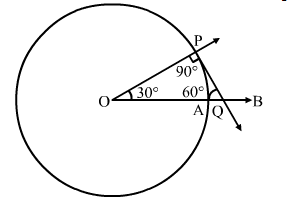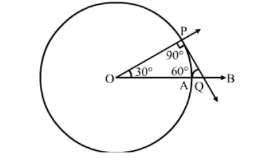# Draw a circle of radius 4 cm.`
Question:

Draw a circle of radius 4 cm. Draw a tangent to the circle, making an angle of 60° with a line passing through the centre.Solution:

Steps Of construction:

Step 1. Draw a circle with centre O and radius 4 cm.
Step 2. Draw radius OA and produce it to B.

Step 3. Make $\angle A O P=30^{\circ}$

Step 4. Draw $\mathrm{PQ} \perp O P$, meeting $\mathrm{OB}$ at $\mathrm{Q}$.

Step 5. Then, $\mathrm{PQ}$ is the desired tangent, such that $\angle O Q P=60^{\circ}$.